# NCERT Solutions Class 5 Mathematics Chapter 13 Ways of Multiply and Divide

## Chapter 13 Ways of Multiply and Divide Class 5 Mathematics NCERT Solutions

Class 5 Mathematics students should refer to the following NCERT questions with answers for Ways of Multiply and Divide in standard 5. These NCERT Solutions with answers for Grade 5 Mathematics will come in exams and help you to score good marks

### Ways of Multiply and Divide Chapter 13 NCERT Solutions Class 5 Mathematics

1. Use Bela’s method to multiply these numbers.

(a) 32 x 46
Ans. (a)
3 2
x 4 6
1 9 2
+ 1 2 8 0
1 4 7 2

(b) 67 x 18
Ans. (b)
6 7
x 1 8
5 3 6
+ 6 7 0
1 2 0 6

2. Do these in your notebook using Bela’s method:

(a) 47 19
Ans. (a)
4 7
x 1 9
4 2 3
+4 7 0
8 9 3

(b) 188 x 91
Ans. (b)
18 8
x 9 1
1 8 8
+1 6 9 2 0
1 4 7 2

(c) 63 x 57

Ans. (c)
6 3
x 5 7
4 4 1
+3 1 5 0
3 5 9 1

(d) 225 x 22
Ans. (d)
22 5
x 2 2
4 5 0
+ 4 5 0 0
4 9 5 0

(e) 360 x 12
Ans. (e)
36 0
x 1 2
7 2 0
+ 3 6 0 0
4 3 2 0

3. Shantaram is a special cook who comes only on party days. Last year he was called for only 28 days. For each day he has to be paid Rs. 165. Find out how much money he will get in all?
Ans. Shantaram’s one day wages = Rs. 165
Shantaram’s 28 days wages = Rs. (165 x 28)
16 5
x 2 8
1 3 2 0
+ 3 3 0 0
4 6 2 0

Hence, Shantaram will be paid Rs. 4620.

4. If he called for all days for the year, how much salary will he get?
Ans. Shantaram’s one day salary = Rs. 165
Shantaram’s one year i.e. 365 days salary = Rs. (165 x 365)
1 6 5
x 3 6 5
8 2 5
+ 9 9 0 0
+ 4 9 5 0 0
6 0 2 2 5

Hence, Shantaram gets a salary of Rs. 60225.

5. Now find the salaries of the minister and horse rider for 1 year.
Ans. For the minister:
Minister’s salary for 1 day = Rs. 195
Minister’s salary for 1 year i.e, 365 days = Rs. (195 x 365)
1 9 5
x 3 6 5
9 7 5
+ 17 7 0 0
+ 5 8 5 0 0
7 1 1 7 5

Hence, the salary of Minister of 1 year is Rs. 71175.
For the horse rider:
Horse rider’s salary for one day = Rs. 76
Horse rider’s salary for 1 year i.e. 365 days = Rs.(76 x 365)
36 5
x 7 6
2 1 9 0
+2 5 5 5 0
2 7 7 4 0

Hence, the salary of horse rider for 1 year is Rs. 27740.

6. Years and Years

(a) How many glasses will he drink in one month?
Ans. (a) Number of glasses of water Sohan drinks in one month i.e. 30 days = (8 x 30) = 240

(b) How many glasses will he drink in one year?
Ans. (b) Number of glasses of water Sohan drinks in one year i.e. 365 days = (8 x 365)
= 2920

(c) If 125 people living in a colony drink 8 glasses of water in a day, how much water will they drink in a year.
Ans. (c) Number of glasses of water a person drinks in 1 day =8
Number of glasses of water 125 persons drink in one day = (8 x 125) = 1000
Number of glasses of water 125 persons drink in one year = (365 x 1000) = 365000

7. If Soha’s heart beats 72 times in one minute, how many times does it beat in one hour.
(a) Now find out how many times it beats in one day.
Ans. (a) Number of times of Soha’s heart beats in 1 minute =72
Number of times Soha’s heart beats in one hour i.e. in 60 minutes = (72 x 60)
= 4320
And Number of times Soha’s heart beats in one day i.e. in 24 hours = (4320 x 24)
We have,
4 3 2 0
x 2 4
1 7 2 8 0
+8 6 4 0 0
1 0 3 6 8 0

(b) Count your heart beats to find out how many times your heart beats in one week?
Ans. (b) On counting I find that my hearts 71 times in one minute.
My heart beats (71 x 60) = 4260 times in one hour
My heart beats (4260 x 24) = 102240 times in a day.
My heart beats (102240 x 7) = 715680 times in one week.

(c) A baby elephant drinks around 1.2 L of milk everyday. How much milk will it drink in two years?
Ans. (c) Number of days in 2 years = (2 x 365) = 730
Milk consumption of baby elephant in 1 day = 12 L
Milk consumption of baby elephant in 2 years i.e. 730 days
73 0
x 1 2
1 4 6 0
+ 7 3 0 0
8 7 6 0

Hence, the baby elephant drinks 8760 L of milk in 2 years.

(d) A baby blue whale drinks around 200 L of milk in one day. Just imagine
how much milk that is! Find out in how many days your family would use 200
L milk. How much milk would the baby blue whale drink in eight months?
Ans. (d) Milk consumption of baby whale per day = 200 L
My family will use 200 L milk at the rate of 20 L per day (200/20) i.e. 10 days
Milk consumption of baby whale in one month i.e. in 30 days = (200 x 30) L = 6000L
Milk consumption of baby whale in 8 months = (6000 x 8) L = 48000 L.

8. Karunya- The Landlord
Karunya bought three fields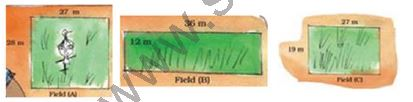(a) Find the area of all the three fields.
Field (A) …. Square metre.
Field (B) …. Square metre.
Field (C) …. Square metre.
He bought field (A) at the rate of Rs. 95 for a square metre , field (B) at Rs.110 for a square metre and field (C) at Rs. 120 for a square metre.
Ans. (a) We know that Area of rectangle = Length Breadth To find the area of fields A, B and C, we have to find 28 x 27, 36 x12 and 27 x 19 respectively.
We have,
2 8
x 2 7
1 9 6
+ 5 6 0
7 5 6

3 6
x 1 2
7 2
+ 3 6 0
4 3 2

(b) Find the cost of all three fields.
Ans. (b) Area of Field (A) = 756 square metre
Area of Field (B) = 432 square metre
Area of Field (C) = 513 square metre
Cost of field (A) at the rate of Rs 95 per square metre = Rs. (95 756) = Rs 71820
7 5 6
x 9 5
3 7 8 0
+ 6 8 0 4 0
7 1 8 2 0

Cost of field (B) at the rate of Rs. 110 per square metre = Rs. (110 x 432) = Rs.47520
1 1 0
x 4 3 2
2 2 0
+ 3 3 0 0
+ 4 4 0 0 0
4 7 5 2 0

Cost of field (C) at the rate of Rs. 120 per square metre = Rs (120 x 513) = Rs. 61560
1 2 0
x 5 1 3
3 6 0
+ 1 2 0 0
+ 6 0 0 0 0
6 1 5 6 0

Total costs of these fields = Rs. 71820 + Rs. 47520 + Rs. 61560
= Rs. 180900

9. Tulsi and her husband work on Karunya’s farm. The Government has said that farm workers should be paid at least Rs 71 for one day’s work. But he pays Rs. 55 to Tulsi and Rs. 58 to her husband.
If Tulsi works for 49 days, how much money does she get?
If her husband works for 42 days, how much money does he get?
Find the money they earn together.
Ans. Tulsi one day’s wage = 55 = Rs. (55 x 49)
5 5
x 4 9
4 9 5
+ 2 2 0 0
2 6 9 5

Hence, Tulsi gets Rs. 2695 for her work.
Husband’s one-day wage = Rs. 58
Husband’s 42 day’s wage = Rs. (58 x 42)
We have,
5 8
x 4 2
1 1 6
+ 2 3 2 0
2 4 3 6

Hence, Tulsi’s husband gets Rs. 2436 for his work.
Money they earned together = Rs. (2695 + 2436) = Rs. 5131

10. The table shows the amount fixed by four states.

(a) For farmwork which state has fixed the highest amount? Which state has fixed the lowest?
Ans. (a) Haryana has fixed the highest salary for one day of the farmworkers whereas Rajasthan has fixed the lowest.

(b) Bhairon Singh is a worker in Rajasthan? If he works for 8 weeks on the
farm, how much will he earn?
Ans. (b)Bhairon Singh’s 1-day earning = Rs. 73
Bhairon Singh’s 1 week earning = Rs. (73 x 7) = Rs. 511
Bhairon Singh’s 8 weeks earning = Rs. (8 x 511) = Rs. 4088

(c) Neelam is a worker in Haryana. If she works for 2(1/2) months on the farm, how much will she earn?
Ans. (c) Number of days in 2(1/2) months = 30 2+ (30/2) = 60 + 15 = 75
Neelam’s 1 day’s earning = Rs. 135
Neelalm’s 2(1/2) months, 75 day’s earning = Rs. (135 x 75)
We have,
1 3 5
x 7 5
6 7 5
+ 9 4 5 0
1 0 1 2 5

Hence. Neelam will earn Rs. 10125.

(d) How much more will a farm worker in Madhya Pradesh get than a worker in Orissa after working for 9 weeks.
Ans. (d) Difference in the salary of workers of Madhya Pardesh and Orissa
= Rs. (97 – 75) = Rs. 22
Difference in their salary in 1 week = Rs. (22 x 7) = Rs. 154
Difference in their salary in 9 weeks = Rs. (154 x 9) = Rs. 1386
Hence, a farmworker in Madhya Pardesh gets Rs. 1386 more than a worker in
Orissa after working for 9 weeks.

11. Satish’s is a 13 year-old boy. His father had taken loan for farming. But the crops failed. Now Satish’s mother has to pay Rs. 5000 every month for the loan. Satish started working- he looked after 17 boats of the village. He earns rupee 1 everyday for one goat.

(a) How much will he earn in one month?
Ans. (a) Satish’s earning on 1 goat per day = Re 1
Satish’s earning on 17 goats per day = Rs (1 x 17) = Rs 17
Satish’s earning on 17 goats in one month i.e. 30 days = Rs (17 x 30) = Rs. 510

(b) Does he earn enough to help pay the loan every month?
Ans. (b) Satish’s earning of 1 year i.e. 12 months = Rs (510 x 12) = Rs 6120

12. To help the farmers the State Government gave cows, Kamla Bai Gudhe got a cow. The cost of the cow is 17500. She had to pay Rs. 5500 and the government spent the rest of the money.
(a) How much did the government spend on the cow?
Ans. Cost of the cow = 17500
Kamla Bai Gudhe had to pay the cost of cow = Rs 5500
Money spend by government = Rs 17500 – Rs 5500 = Rs 12000
Hence, the government spend Rs 12000 on the cow.''

(b) If 9 people from her village got cows, how much did the government spend in all?
Ans. (b) The amount of money spent by the government on 9 cows = Rs (12000 x 9) = Rs 108000.'

(c) If Kamla Bai spends Rs. 85 a day, find out how much she will spend in one month?
Ans. (c) Amount spent by Kamla Bai in 1 day = Rs 85
Amount spent by Kamla Bai in 1 month = Rs (85 x 30) = Rs 2550'

(d) The cow gives 8 liters of milk everyday. How much milk it give in one month?
Ans. (d) Quantity of milk given by cow in 1 day = 8 litres
Quantity of milk given by cow in 1 month = (8 x 30) litres = 240 litres'

(e) If the milk is sold at Rs. 9 per litre, how much money will Kamla Bai make in one month?
So the money spent on keeping the cow was Rs. …..
Money earned by selling the milk Rs. …….
Which is more …. Money spent on the cow or money earned from it? How
much?
Ans. (e) Money earned by selling the milk = Rs. (240 x 9) = Rs 2160
Since 2550 > 2160
Therefore, money spent on cow was more than the money earned. The difference
was Rs (2550-2160) = Rs 390
Due to this loss she wanted to sell the cow.

13. (a) Sukhi works on a farm. He is paid Rs. 98 for one day. If he works for 52 days, how much will he earn?
Ans. (a) Sukhi’s 1-day earning = Rs. 98
= Rs (98 x 52) = Rs 5096
9 8
x 5 2
1 9 6
+4 9 0 0
5 0 9 6

Hence, Sukhi earns Rs 5096 in 52 days.

(b) Hariya took a loan to build his house. He has to pay back Rs 2750 every month for two years. How much will he pay in 2 years?
Ans. (b) Amount of loan paid back in 1 month = Rs. 2750
Amount of loan paid back in 2 years i.e. (2 x 12 = 24 months) = Rs. (2750 x 24)
= Rs. 66000
2 7 5 0
x 2 4
1 1 0 0 0
+ 5 5 0 0 0
6 6 0 0 0

(c) Ratiram is a milk seller in the city. He sells 13 litres of milk every day at Rs ​​​​​​​23 per litre. How much does he earnb?
Ans. Selling price of 1 litres of milk = Rs. 23
Selling price of 13 litres of milk = Rs. (23 x 13) = Rs. 299

(d) A farmer sells 1 litres of milk for Rs. 11. In one month he sells 210 litres of milk. How much does he earn in a month?
Ans. (d)Selling price of 1 litres of milk = Rs. 11
Selling price of 210 litres of milk = Rs. (210 x 11) = Rs. 2310

(e) A company sells 1 litre of packed water for Rs. 12. A shopkeeper buys 240 litres of packed water. How much does he pay?
Ans. (e)Amount paid for 1 litre of packed water = Rs. 12
Amount paid for 240 litres of packed water = Rs. (12 x 240) = Rs. 2880

14. Fun with Multiplication:
(A) Look for the pattern and take this forward.
(0 x 9) + 1 = 1
(1 x 9) + 2 = 11
(12 x 9) + 3 = 111
(123 x 9) + 4 = _______
(1234 x 9) + 5 = ________
(12345 x 9) + 6 = _________
Ans. (A)(123 x 9) + 4 = 1107 + 4 = 1111
(1234 x  9) + 5 = 11106 + 5 = 11111
(12345 x 9) + 6 = 11105 + 6 =111111
(B) Each letter a, b, c here stands for a number.
a a a
a a a
a a a

a a a 0
a a a 0 0
a b c b a
Taking a =1, then find what the number b and c will be.
Ans. (B)Taking a = 1, so we have to compete 111 111 to find the value of b and c.
1 1 1
x 1 1 1
1 1 1
1 1 1 0
1 1 1 0 0
1 2 3 2 1

On Comparing the answers, we find b = 2 and c = 3.

Multiply it by 7 _____
Again multiply the answer by 13 _____
Ans. (C) Let be my age by 9 years
On multiplying 9 by 7, we get 63.
Again, multiply 63 by 13, we get 819
Yes, I find my age in the final result. My age occurs two times in the answer.

15. Practice Time:
(a) 4228 ÷ 4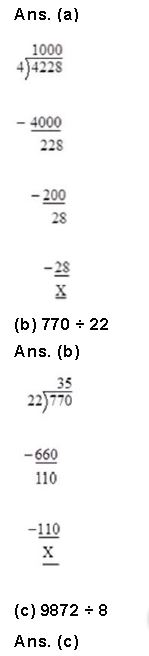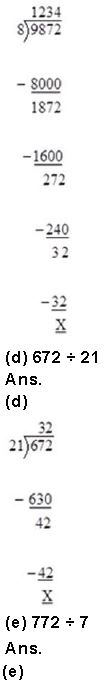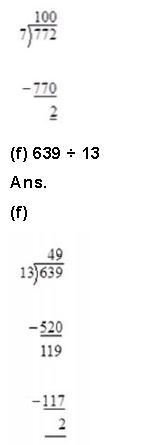16. Isha has Rs 100 with her. She wants to buy petrol. One litre of petrol costs Rs. 47.ow How How many litres can she buy?
Ans. Money with Isha = Rs. 1000
Cost of one litre = Rs. 47
Litres of petrol she can buy = 1000÷47 = 21 (Quotient), 13 (Remainder)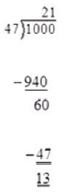17. If Is ha comes to your city, how much petrol can she buy with the same money?
Ans.
Money left with Isha = Rs. 1000
Cost of 1 litres of petrol in the city = Rs. 50
Litres of petrol she can buy = Rs. 1000 ÷ 50 = 20
Isha can buy 20 litres of petrol for the same money.

18. 576 books are to be packed in boxes. If one box has 24 books, how many boxes are needed?
Ans. Total number of books = 576
Number of books per box = 24
Number of boxes needed or packing = 576 ÷ 24 = 24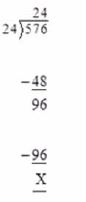19. 836 people are watching a movie in a hall. If the hall has 44 rows, how many people can sit in 1 row?
Ans. Number of people watching a movie = 836
Number of rows = 44
Number of people sitting in 1 row = 836 ÷ 44 = 1920. A gardener bought 458 apples trees. He wants to plant 15 trees in each row. How many rows can he plant?
Ans.
Number of trees bought = 458
Number of trees planted in 1 row = 15
Number of rows = 458 ÷ 15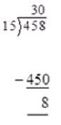We get 30 as quotient and 8 as remainder.
Hence, he can plant in 30 rows and 8 trees are left over.

21. Shyamli bought a battery. She road on it ‘Life: 2000 hours’. She uses it throughout the day and the night. How many days will the battery run?
Ans.
Life of battery = 2000 hours
The number of days the battery will run = 2000÷ 24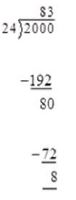We get 83 as quotient and 8 as remainder. Hence, the battery will run for 83 days.

22. A tank is full of 300 L of water. How much water will be filled in 25 tanks?
If 15 buckets can be filled with one tank of water, how many buckets all can be filled with the water in 25 tanks?
Ans.
Capacity of water tank = 300 L
25 tanks can be filled with (25 x 300) L i.e. 7500 L of water.
Number of buckets that can be filled with 1 tank = 15
Number of buckets that can be filled with 25 tanks = (15 x 25) =375

23. There are 28 laddoos in 1 kg. How many laddoos will be there in 12 kg? If 16 laddoos can be packed in 1 box, how many boxes are needed to pack all these laddoos?
Ans. Number of laddoos in 1 kg = 28
Number of laddoos in 12 kg = 28 x 12 = 336
Total number of laddoos = 336
Number of laddoos packed in 1 box = 16
Number of boxes needed for packing = 336 ÷ 16 = 2124.There are 26 rooms in a school. Each room has 4 plant. If each plant needs 2 cups of water, how much water do we need for all the plants?
Ans.
Number of rooms in a school = 26
Number of plants per room = 4
Total number of plants = 26 x 4 = 104
Water needed for each plant = 2 cups
Water needed for 104 plants = 104 x 2 = 208 cups

25. Do these divisions. Check your result by multiplication.
(a) 438 ÷ 9
Ans.
(a)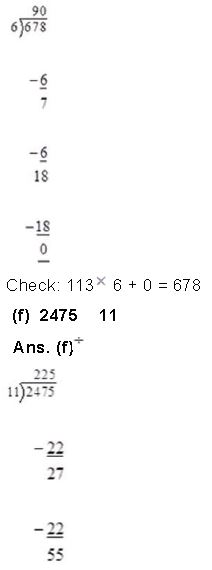Check: 225 11 + 0 =2475

26. Solve the given sums and colour the answers in the grid given below: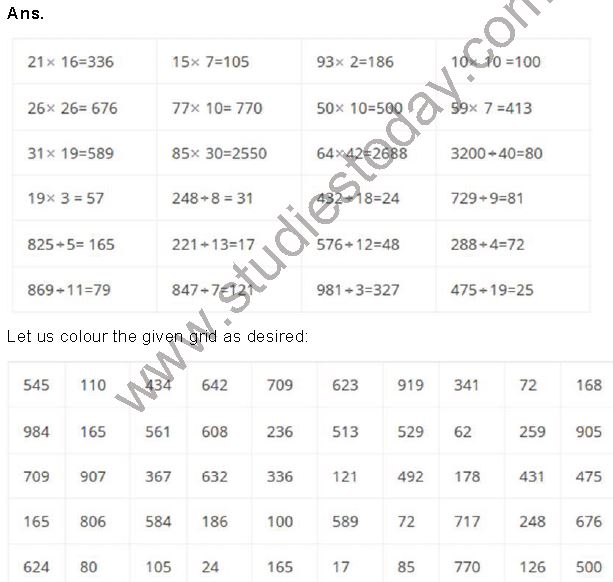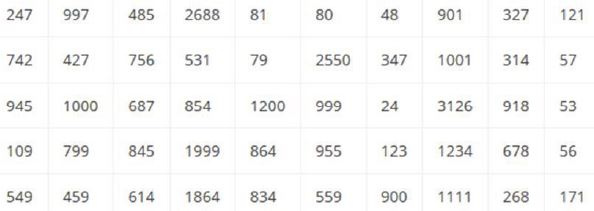NCERT Solutions Class 5 Mathematics Chapter 1 The Fish Tale
 NCERT Solutions Class 5 Mathematics Chapter 2 Shapes and Angles
 NCERT Solutions Class 5 Mathematics Chapter 3 How Many Squares
 NCERT Solutions Class 5 Mathematics Chapter 4 Parts and Wholes
 NCERT Solutions Class 5 Mathematics Chapter 5 Does it look the same
 NCERT Solutions Class 5 Mathematics Chapter 6 Be my multiply
 NCERT Solutions Class 5 Mathematics Chapter 7 Can you see the pattern
 NCERT Solutions Class 5 Mathematics Chapter 8 Mapping Your Way
 NCERT Solutions Class 5 Mathematics Chapter 9 Boxes And Sketches
 NCERT Solutions Class 5 Mathematics Chapter 10 Tenths And Hundredths
 NCERT Solutions Class 5 Mathematics Chapter 11 Area and its Boundary
 NCERT Solutions Class 5 Mathematics Chapter 12 Smart Charts
 NCERT Solutions Class 5 Mathematics Chapter 13 Ways of Multiply and Divide
 NCERT Solutions Class 5 Mathematics Chapter 14 How Big How Heavy

Tags